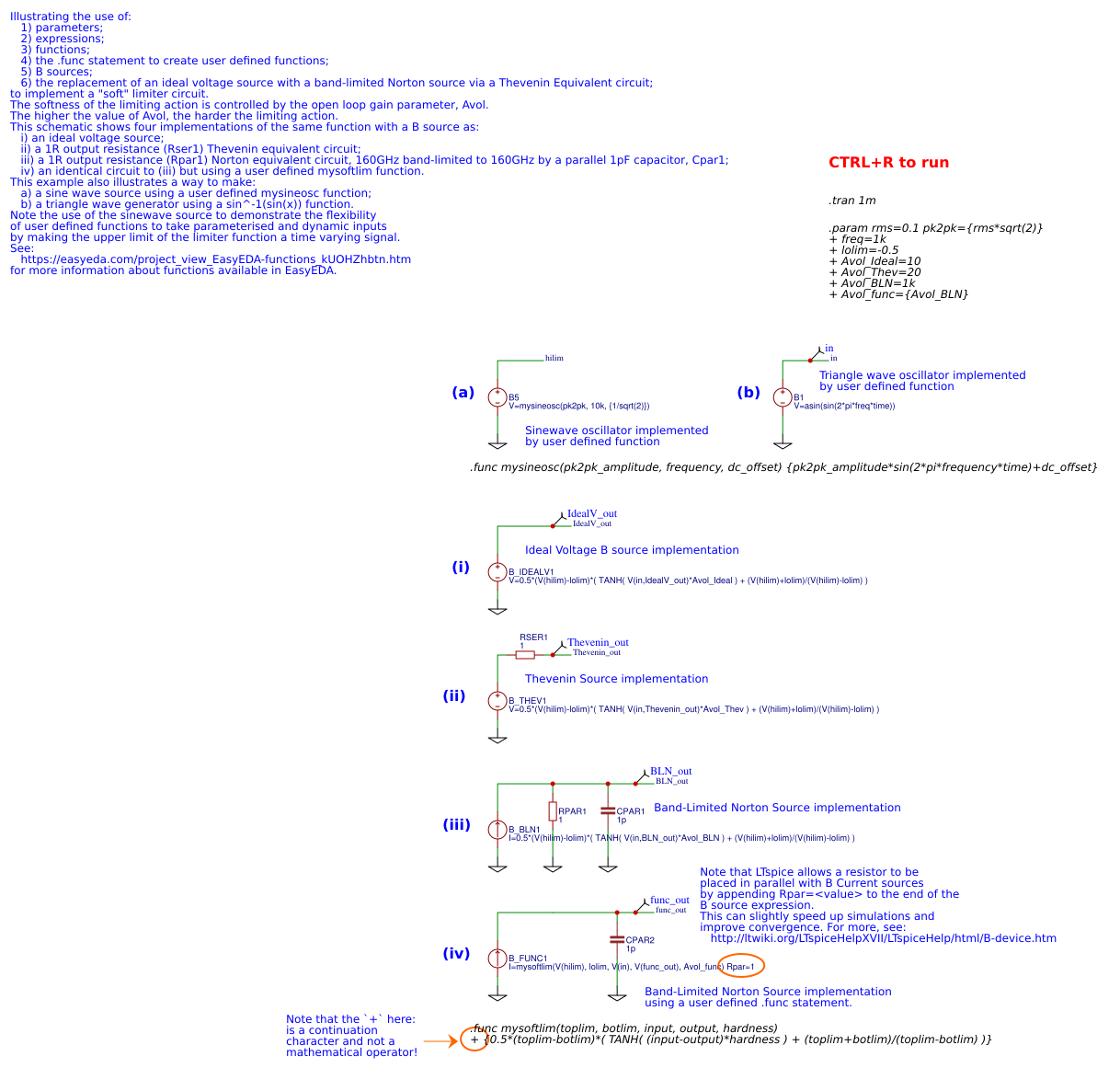Public Domain

Recommend Projects

# Parameters, expressions, functions and B Sources

2 years ago 432
• Description
• Documents
• BOM
• Attachments
• Members

## Description

Illustrating the use of:

1) parameters;

2) expressions;

3) functions;

4) the .func statement to create user defined functions;

5) B sources;

6) the replacement of an ideal voltage source with a band-limited Norton source via a Thevenin Equivalent circuit;

to implement a "soft" limiter circuit.

The softness of the limiting action is controlled by the open loop gain parameter, Avol.

The higher the value of Avol, the harder the limiting action.

This schematic shows four implementations of the same function with a B source as:

i) an ideal voltage source;

ii) a 1R output resistance (Rser1) Thevenin equivalent circuit;

iii) a 1R output resistance (Rpar1) Norton equivalent circuit, 160GHz band-limited to 160GHz by a parallel 1pF capacitor, Cpar1;

iv) an identical circuit to (iii) but using a user defined `mysoftlim` function.

This example also illustrates a way to make:

a) a sine wave source using a user defined `mysineosc` function;

b) a triangle wave generator using a sin^-1(sin(x)) function.

Note the use of the sinewave source to demonstrate the flexibility of user defined functions to take parameterised and dynamic inputs by making the upper limit of the limiter function a time varying signal.

See:

http://easyeda.com/project_view_EasyEDA-functions_kUOHZhbtn.htm

## Parameters, expressions, functions and B Sources## BOM

ID Name Designator Quantity
1 I=0.5*(V(hilim)-lolim)*( TANH( V(in,BLN_out)*Avol_BLN ) + (V(hilim)+lolim)/(V(hilim)-lolim) ) B_BLN1 1
2 V=0.5*(V(hilim)-lolim)*( TANH( V(in,IdealV_out)*Avol_Ideal ) + (V(hilim)+lolim)/(V(hilim)-lolim) ) B_IDEALV1 1
3 V=asin(sin(2*pi*freq*time)) B1 1
4 V=0.5*(V(hilim)-lolim)*( TANH( V(in,Thevenin_out)*Avol_Thev ) + (V(hilim)+lolim)/(V(hilim)-lolim) ) B_THEV1 1
5 1 RSER1,RPAR1 2
6 1p CPAR1,CPAR2 2
7 I=mysoftlim(V(hilim), lolim, V(in), V(func_out), Avol_func) Rpar=1 B_FUNC1 1
8 V=mysineosc(pk2pk, 10k, {1/sqrt(2)}) B5 1

## Attachments

None
Success
The owner does not allow comments in this project now